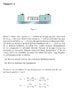# Elastic collision with two masses and spring

• eventob

## Homework Statement

Had to translate the original problem statement in order to post it here; sorry about the grammar.

Block 1 has a mass m1=2.00kg, moving to the right (along the x-axis) with an initial velocity vi1=10.0 m/s. Block 2 has a mass m2=5.00kg and is moving in the same direction as block 1, with an initial velocity v2i = 3.00 m/s. The surface is frictionless.

Block 2 is equipped with an ideal spring with Ks=1.12 kN/m.

When the two blocks collide, the spring will first compress, then expand to it's equilibrium position. The masses will then move independently.

The compression of the springs will have it's greatest value when the masses are moving with uniform velocity.

The collision is elastic.

Find the maximum compression of the spring.

## Homework Equations

I know that when we model the collision as elastic, both the kinetic energy and the linear momentum of the system is preserved.

delta P=0
delta k = 0

## The Attempt at a Solution

Tried to use the formulas above, and solve the system of equations. The problem would be very easy to solve, without the spring.

#### Attachments

•hmmmmm.jpg
28.7 KB · Views: 2,791
Hint: How fast are the masses moving when the spring is at maximum compression? How can you figure that out?

The masses will have the same velocity at the instant where the spring is at maximum compression? I guess I could use the formula for conservation of linear momentum

M1*V1i+M2*V2i=M2*V2f+M1*V1f

If I make the assumption that the speed of the masses is the final speed right after the collision, I'll end up with something like this:
M1*V1i+M2*V2i=(M1+M2)Vf

If the kinetic energy of the system is constant:

(1/2)*m1*v1f-(1/2)*m1v1i+(1/2)*m2*v2f-(1/2)*m2v2i+(1/2)kx^2=0

But I don't think the last equation is correct?

Thanks for helping me out. :)

The masses will have the same velocity at the instant where the spring is at maximum compression?
Exactly! That's the key.
I guess I could use the formula for conservation of linear momentum

M1*V1i+M2*V2i=M2*V2f+M1*V1f

If I make the assumption that the speed of the masses is the final speed right after the collision, I'll end up with something like this:
M1*V1i+M2*V2i=(M1+M2)Vf
Right! Vf is the speed at the point of maximum compression.

If the kinetic energy of the system is constant:

(1/2)*m1*v1f-(1/2)*m1v1i+(1/2)*m2*v2f-(1/2)*m2v2i+(1/2)kx^2=0

But I don't think the last equation is correct?
You forgot to square the speeds in the KE terms: ½mv². Also, v1f = v2f = Vf.

If I use the first equation derived from the cinservation of linear momentum, could I solve for the spring compression like this:

Right before collision:

Emek_i=K1+K2+Us

Where K1 is the kinetic energy of mass 1, K2 is the kinetic energy if mass 2 and Us is the potential energy of the spring (which is zero for now)

Right after the collision:
Emek_f=K+Us

Where K is the compound kinetic energy of the two masses, and Us is the elastic energy stored in the spring (1/2 k*x^2) ?

Unfortunately, I don't have the answer to this problem, I got it from my mechanics professor today, It' not from the text-book, and I can't find any examples realting to it.

Edit: Didn't see your last answer before I posted. Thanks, think I managed to solve the problem now. Thanks. :D

You've got it exactly right.

Got a follow-up question. Just found the maximum compression of the spring to be 0.267m. In part b of the problem, I am supposed to figure out the final speed of the masses.

If you look at the attachement in the original post, there is two equations there. Is this just a simple substitution problem? When i substitute the numerical data into the equations, i get V1f= 0, and V2f=6.57 m/s.

My intuition tells me that mass 1 should accelerate in the negative direction, opposite of it's initial direction, due to the force excerted by the spring.

If you look at the attachement in the original post, there is two equations there. Is this just a simple substitution problem?
Yes, since they give you the formulas for the final speeds after an elastic collision. (Usually you need to derive those results, which is a pain.)
When i substitute the numerical data into the equations, i get V1f= 0, and V2f=6.57 m/s.
I didn't confirm your arithmetic, but I don't see any problems.

My intuition tells me that mass 1 should accelerate in the negative direction, opposite of it's initial direction, due to the force excerted by the spring.
Your intuition is correct. Mass 1 started out with a speed of +10.0 m/s and it ends up with zero speed--sounds like a negative acceleration to me.